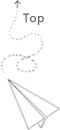# OpenGL 筆記 - Coordinate System

OpenGL 座標的值在 經過 Vertex Shader 之後變成 $[-1.0, 1.0]$ 的座標，稱作 標準化設備座標 Normalized Device Coordinates (NDC)，只有在此座標內的頂點最終才會顯示在螢幕上。

• 座標系統
• 局部空間 Local Space 或是 物體空間(Object Space)
• 世界空間 World Space
• 觀察空間 View Space 或 Eye Space
• 裁剪空間 Clip Space
• 螢幕空間 Screen Space• Local Space
• 物體相對於局部原點的座標
• World Space
• 相對於世界的原點，會和其他物體相對於原點擺放
• View Space
• 從 Camera (觀察者) 的角度進行觀察的頂點座標
• Clip Space
• View Space 經過投影後，裁剪座標會被處理到 NDC 空間 $[-1.0, 1.0]$
• Screen Space
• 經過 Viewport Transform，將 $[-1.0, 1.0]$ 內的座標變換到 glViewport 所定義的座標範圍內
• 出來的座標會送到 Rasterizer 變成 Fragment (Pixel)

## Clip Space 裁剪空間### 正射投影 Orthographic Projectionmatrix 長相

$\begin{pmatrix} \frac{2}{\text{right}-\text{left}} & 0 & 0 & t_x \\ 0 & \frac{2}{\text{top} - \text{bottom}} & 0 & t_y \\ 0 & 0 & \frac{2}{\text{zFar} - \text{zNear}} & t_z \\ 0 & 0 & 0 & 1 \end{pmatrix}$
$t_x = - \frac{\text{right}+\text{left}}{\text{right}-\text{left}} \\ t_y = - \frac{\text{top} + \text{bottom}}{\text{top} - \text{bottom}} \\ t_z = - \frac{\text{zFar} + \text{zNear}}{\text{zFar} - \text{zNear}}$

### 透視投影 Perspective Projection• Perspective Division 透視除法
• 在轉換到 Clip Space 的頂點，每個座標的 $(x, y, z)$ 會都除上 $w$ 分量
• 也就是 $(\frac{x}{w}, \frac{y}{w}, \frac{z}{w})$
• 將 4D 的 Clip Space 座標轉換成 3D NDC 座標
• 在 Vertex Shader 的最後會被 自動執行### 比較## 組合在一起

$V_{clip} = M_{projection} \cdot M_{view} \cdot M_{model} \cdot V_{local}$

## 進入 3D 世界

• 右手坐標系
• OpenGL 的座標系• 那我們想要把 Camera 擺在 $(0, 0, 3)$ 然後往 $-z$ 方向看要怎麼辦?
• 我們不能移動 Camera
• 那就移動物體，往相反於 Camera 移動的方向，移動整個場景
• View Matrix## Examples• 3D 的立方體
• 要畫一個立方體需要 36 個頂點
• 改用 glDrawArrays() 因為沒有 EBO
• 6.2.cube• 啟用 Depth Test

• 每一 frame 都要清掉 Z-Buffer 的內容• 顯示更多的立方體
• 每次畫一個立方體時，就算出一個 Model Matrix

## Labs

• 將觀察矩陣在各個方向上進行位移，來看看場景是如何改變的。• 對 GLM 的projection函數中的 FoV 和 aspect-ratio 參數進行實驗。看能否搞懂它們是如何影響透視 frustum 的。#### 參考

https://blog.csdn.net/chy19911123/article/details/45476821

## 推薦文章